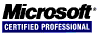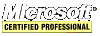# Intel IA-32 Assembly Tutorial

#### Data-Related Operators and Directives

`OFFSET` operator returns the offset of a data label. The offset represents the distance in bytes of the label from the beginning of the data segment.

``````	.data
byt BYTE ?
wrd WORD ?
dbl DWORD ?
array WORD 1,2,3,4,5,6,7,8,9
.code
mov esi,OFFSET byt       ;assume byt located at 00808000h = ESI
mov esi,OFFSET wrd       ;00808001h = ESI
mov esi,OFFSET dbl       ;00808003h = ESI
mov esi,OFFSET array + 6 ;0080800Dh = ESI
``````

The `ALIGN` directive is used to align a variable on an even-numbered address in memory because the CPU can access these variables faster.

``````
.data
byt BYTE ?       ;00808000h
ALIGN 2
wrd WORD ?       ;00808002h
dbl DWORD ?      ;00808004h
``````

Use the `PTR` directive to override the default size of an operand.

``````	.data
dbl DWORD 12345678h
wdbl WORD 5678h,1234h
.code
mov al,BYTE PTR dbl     ;al = 78h
mov al,BYTE PTR [dbl+1] ;al = 56h
mov al,BYTE PTR [dbl+2] ;al = 34h
mov al,BYTE PTR [dbl+3] ;al = 12h
mov eax,DWORD PTR wdbl  ;eax = 12345678h
``````

The `TYPE` operator returns the size in bytes of a single element of a variable.

The `LENGTHOF` operator returns the number of elements in an array.

Using the `SIZEOF` operator is the same as multiplying `LENGTHOF` by `TYPE`.

The `LABEL` directive can be used to construct a larger integer from to smaller ones and vice-versa.

``````	.data
wordword LABEL DWORD
var1 WORD 5678h
var2 WORD 1234h
.code
mov eax,wordword ;EAX = 12345678h
``````

By using a register as a pointer to access an array is called indirect addressing.

``````	.data
val BYTE Fh
.code
mov esi,OFFSET val
mov al,[esi]       ;al = Fh, [esi] is a pointer and this only works on registers
``````

The assembler does not know whether `ESI` points to a BYTE, WORD or DWORD. The `PTR` operator tells the assembler what a registry points to. Note: EAX, EBX, ECX, EDX, etc. could have been used with the same results.

``````	inc [esi]		;DOES NOT WORK!
inc BYTE PTR [esi]
``````

Indirect operands are needed to deal with arrays.

``````	.data
bytearray BYTE 1,2,3,4,5,6,7,8
wordarray WORD 10,11,12,13,14,15,16
.code
mov esi,OFFSET bytearray
mov al,[esi]    ;AL = 1
add esi,1       ;increment by 1 (byte)
mov al,[esi]    ;AL = 2
add esi,1       ;increment by 1 (byte)
mov al,[esi]    ;AL = 3

mov esi,OFFSET wordarray
mov al,[esi]    ;AL = 10
add esi,2       ;increment by 2 (bytes)
mov al,[esi]    ;AL = 11
add esi,2       ;increment by 2 (bytes)
mov al,[esi]    ;AL = 12
``````

An indexed operand adds a constant to a register to generate an effective address.

``````	.data
bytearray BYTE 1,2,3,4,5,6,7,8
wordarray WORD 10,11,12,13,14,15,16
.code
mov esi,OFFSET bytearray
mov al,[esi]     ;AL = 1
mov al,[esi+1]   ;AL = 2
mov al,[esi+2]   ;AL = 3

mov esi,OFFSET wordarray
mov al,[esi]     ;AL = 10
mov al,[esi+2]   ;AL = 11
mov al,[esi+4]   ;AL = 12
``````

A variable that contains the address of another variable is a pointer.

``````	.data
bytearray BYTE "Hello World!",0
ptrbyte DWORD OFFSET bytearray
``````

This little code snippet demonstrates loading the address of a variable that points to a `DWORD` into the `ESI` register. It then increments the variable pointed to by one and then moves that value into the `EAX` register.

``````	mov esi,[esp+8]
inc DWORD PTR [esi]
mov eax,DWORD PTR [esi]
``````

The `TYPEDEF` operator creates a user-defined type. `TYPEDEF` is useful for creating pointer variables. This example creates new data types that are pointers:

``````	PBYTE TYPEDEF PTR BYTE
PWORD TYPEDEF PTR WORD
PDWORD TYPEDEF PTR DWORD
``````

The `JMP` instruction jumps unconditionally to a code label and the `LOOP` instruction repeats a block of statements a specified number of times. A `LOOP` instruction must jump between -128 to +127 bytes. Instructions have an average size of 3 bytes.

``````	.data
.code
lblBegin:
xor edx,edx     ;edx = 0
mov ecx,10      ;ecx is used by LOOP as a counter
lblLoop:
inc edx
loop lblLoop    ;first subtract 1 from ecx then if ecx is zero stop looping otherwise jump to lblLoop
jmp lblBegin    ;jmp unconditionally to the label lblBegin
``````

Example copying a string from a source to a destination using the `LOOP` instruction:

``````	.data
sour BYTE "copy me to a new place in memory.",0
dest BYTE SIZEOF sour DUP(0),0
.code
xor esi,esi
mov ecx,SIZEOF sour
lblLoop:
mov al,sour[esi]
mov dest[esi],al